# Effect of in-wheel motor suspension system on electric vehicle ride comfort

## Le Van Quynh1, Bui Van Cuong2, Nguyen Van Liem3, Le Xuan Long4, Pham Thi Thanh Dung5

1, 2, 3, 4Faculty of Automotive and Power Machinery Engineering, Thai Nguyen University of Technology, Thai Nguyen, Vietnam

5Ministry of National Defense, The Vocational College No. 1, Thai Nguyen, Vietnam

1Corresponding author

Vibroengineering PROCEDIA, Vol. 29, 2019, p. 148-152. https://doi.org/10.21595/vp.2019.21175
Received 4 November 2019; accepted 11 November 2019; published 28 November 2019

43rd International Conference on Vibroengineering in Greater Noida (Delhi), India, November 28-30, 2019

Copyright © 2019 Le Van Quynh, et al. This is an open access article distributed under the Creative Commons Attribution License, which permits unrestricted use, distribution, and reproduction in any medium, provided the original work is properly cited.
Views 765
Abstract.

This paper presents the effect of in-wheel motor (IWM) suspension system on electric vehicle (EV) ride comfort. To analyze the impact on the vehicle ride comfort caused by IWM suspension system, a dynamic model of quarter vehicle is established with the combination of IWM and road surface roughness excitations. The weighted root-mean-square (r.m.s.) acceleration of the vertical vehicle body (${a}_{wbz}$) according to the international standard ISO 2631-1 (1997) is selected as an objective function to analyze the effect of IWM suspension system when the road surface and IWM mass conditions change. The study results indicate that the effect of IWM suspension system on the EV ride quality is significant and the value of ${a}_{wbz}$ reduces by 8.6 % in comparison to without IWM suspension system. The IWM suspension system has significantly improved the EV ride quality when the road surface and IWM mass conditions change.

Highlights
• In-wheel motor (IWM) suspension system
• Electric Vehicle (EV)
• The combination of IWM and road surface roughness excitations
• Electric vehicle ride comfort

Keywords: electric vehicle, in-wheel electric motor, suspension system, dynamic model, ride quality.

#### 1. Introduction

In recent years, in order to reduce environmental pollutions, electric vehicles have been studied and developed by researchers to improve the vehicle efficiency and they also pay much attention to perfecting the design to improve the ride quality. Yechen Qin et al. proposed a new approach for vibration mitigation based on a dynamic vibration absorbing structure (DVAS) for electric vehicles (EVs) using a model of the coupled longitudinal-vertical dynamics . Abu Bakar S. A. et al. presented a simulation study on the ride comfort performance of a passenger vehicle which is expected to be converted into an electric vehicle using a validated 7 degrees of freedom of vehicle ride comfort model of the passenger vehicle . Liqiang Jin et al. analyzed the influence of the ratio between unsprung and sprung mass on ride comfort of vehicles driven by in-wheel motors using an 11 degrees of freedom of vehicle ride comfort model .

To improve the electric vehicle ride comfort, the optimization, control, and optimal control methods are used to control EV suspension system. Abdussalam Ali A. O. and Başar Özkan analyzed the effect of in-wheel electric motors mass on the performance of passive and active suspension systems . A modified GPSO-LQG controller is proposed by Fangwu Ma et al. for one-quarter EV suspension with the purpose of optimizing suspension performance for entire speed ranges . Liu M. et al. proposed the ride comfort optimization of In-Wheel-Motor EV with In-Wheel vibration absorbers using two control methods such as the linear quadratic regulator (LQR) algorithm for suspension damper and the fuzzy PID method for in-wheel damper . Ma Y. et al. proposed a new electric wheel with active control of suspension using LQG control theory for in-wheel motor . Di Tan et al. proposed a dual-loop proportion integration differentiation controller based on the particle swarm algorithm is designed to control the active suspension of the EV driven by in-wheel motor .

The EV dynamic models are applied to study vehicle suspension and in-wheel electric motor suspension such as the quarter EV dynamic model [1, 7, 9], the half EV dynamic model  and the full vehicle [2, 3]. Vibration sources are considered excitation inputs for the EV dynamic models such as road surface [2, 3], in-wheel electric motor as well as the association of both excitation sources .

The major goal of this study is to establish a dynamic model of quarter vehicle with the combination of IWM vertical exciting force and road surface roughness excitations to analyze the effect of IWM suspension system on EV ride comfort. The ${a}_{wbz}$ value according to the international standard ISO 2631-1 (1997)  is selected as an objective function to analyze the effect of IWM suspension system when the road surface and IWM mass conditions change.

#### 2.1. One-quarter EV dynamic model

A one-quarter EV dynamic model without IWM suspension system and with IWM suspension system were established for analyzing the effect of IWM suspension system on EV ride comfort, as shown in Fig. 1.

Fig. 1. One-quarter electric vehicle dynamic model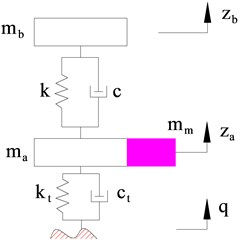a) Without IWM suspension system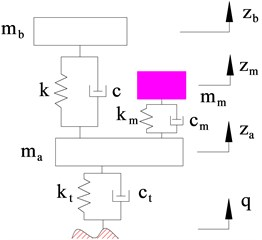b) With IWM suspension system

In Fig. 1, ${m}_{b}$, ${m}_{m}$ and ${m}_{a}$ are the sprung mass of EV body, IWM mass, and EV unsprung mass respectively; ${k}_{t}$ and ${c}_{t}$ are the stiffness and damping coefficients of the tire; $k$and $c$ are the stiffness and damping coefficients of the EV suspension system; ${k}_{m}$ and ${c}_{m}$ are the IWM suspension system; ${z}_{a}$, ${z}_{b}$ and ${z}_{m}$ are the vertical displacements of the axles, vehicle body and IWM mass, respectively; $q$ is road surface excitation

Equations of motion: From the EV dynamic model as shown in Fig. 1(b), the dynamic equation of the quarter vehicle using Newton’s second law are written as follows:

(1)
${m}_{b}{\stackrel{¨}{z}}_{b}=-\left[k\left({\mathrm{z}}_{b}-{z}_{a}\right)+c\left({\stackrel{˙}{\mathrm{z}}}_{b}-{\stackrel{˙}{z}}_{a}\right)\right],$
(2)
${m}_{m}{\stackrel{¨}{z}}_{m}={F}_{mz0}-\left[{k}_{m}\left({\mathrm{z}}_{m}-{z}_{a}\right)+{c}_{m}\left({\stackrel{˙}{\mathrm{z}}}_{m}-{\stackrel{˙}{z}}_{a}\right)\right],$
(3)
${m}_{a}{\stackrel{¨}{z}}_{a}=\left[k\left({\mathrm{z}}_{b}-{z}_{a}\right)+c\left({\stackrel{˙}{\mathrm{z}}}_{b}-{\stackrel{˙}{z}}_{a}\right)\right]+\left[{k}_{m}\left({\mathrm{z}}_{m}-{z}_{a}\right)+{c}_{m}\left({\stackrel{˙}{\mathrm{z}}}_{m}-{\stackrel{˙}{z}}_{a}\right)\right]$

where, ${F}_{mz0}$ is IWM vertical exciting force.

#### 2.2.1. Road surface excitation model

Many studies have shown there are several types of single-point time domain models of road irregularity excitation, namely FFT, AR/ARM, white noise filtering  and harmony superposition  methods. Based on the study carried out by Dodds C. J. and Robson J. D. , the road surface roughness is usually assumed to be a zero-mean stationary Gaussian random process and can be generated through an inverse Fourier transformation based on a power spectral density (PSD) function. The time domain excitation of the uneven road surface is generated as the sum of a series of harmonics:

(4)
$q\left(t\right)={\sum }_{k=1}^{N}\sqrt{2{G}_{q}\left({f}_{mid-k}\right)\mathrm{\Delta }{f}_{k}}\mathrm{s}\mathrm{i}\mathrm{n}\left(2\pi {f}_{\mathrm{m}\mathrm{i}\mathrm{d}-k}t+{\phi }_{k}\right),$

where, ${G}_{q}\left({f}_{mid-k}\right)$ is power spectrum, m2/Hz; $∆f$ is the frequency range, Hz; $t$ is time, s; ${\phi }_{k}$ is the random phase uniformly distributed from 0 to 2$\pi$.

#### 2.2.2. IWM vertical exciting force

Yuewei Yu et al. (2019) proposed a nonlinear dynamic model of an electric vehicle with the in-wheel motor driving system which is established on the basis of considering the motor exciting force and the bearing nonlinear force . The nonlinear forces of the bearing in the $X$ direction and $Z$ direction are defined as:

(5)
${F}_{x}={\sum }_{j=1}^{N}{F}_{j}\mathrm{c}\mathrm{o}\mathrm{s}{\theta }_{j},$
(6)
${F}_{z}={\sum }_{j=1}^{{N}_{b}}{F}_{j}\mathrm{s}\mathrm{i}\mathrm{n}{\theta }_{j},$

where, ${\theta }_{k}$ is the angular position of the $j$th rolling element.

In this study, we only consider the effect of the motor's vertical force, Eq. (6) can be rewritten as:

(7)
${F}_{mz0}={m}_{s}e{\omega }_{R}^{2}\mathrm{c}\mathrm{o}\mathrm{s}{\omega }_{R}t,$

where, ${m}_{s}$ is the total mass of the tyre, the rim and the motor rotor; $e$ is the eccentricity of the rotor; ${\omega }_{R}$ is the angular velocity of the rotor.

#### 3. Results and discussion

The differential equations of the quarter vehicle in Section 2 are solved based on the MATLAB/Simulink with a set of parameters of EV by the references . When vehicle moves on the ISO road surface class B (according to the International Standards Organization CISO 8608 ) at vehicle speed $v$ = 50 km/h and IWM vertical exciting force ${F}_{mz0}=1500\mathrm{c}\mathrm{o}\mathrm{s}\left(1000t\right)/N$ at two cases such as with IWM suspension system in comparison without IWM suspension system (Case 1) and with IWM vertical exciting force in comparison without IWM vertical exciting force (Case 2), the simulation result of the time domain responses of the vehicle body vertical acceleration is shown in Fig. 2.

From the results of Fig. 2(a), we could determine that according to the international standard ISO 2631-1 the values of the weighted root-mean-square (r.m.s.) acceleration of the vertical vehicle body (${a}_{wbz}$) are 0.417 m/s2 without IWM suspension system and 0.381 m/s2 with IWM suspension system, respectively with case 1. The IWM suspension system makes the value of ${a}_{wbz}$ reduce by 8.6 % in comparison without IWM suspension system which means that the effect of IWM suspension system on the vehicle ride quality is significant. Similarly, from the results of Fig. 2(b), ${a}_{wbz}$ the value 0.381 m/s2 with IWM vertical exciting force and 0.369 m/s2 without IWM vertical exciting force. The IWM vertical exciting force makes the value of ${a}_{wbz}$ increase by 2.2 % in comparison without IWM vertical exciting force.

Comparing with the IWM suspension system and without IWM suspension system when vehicle moves under the different operating conditions: the ${a}_{wbz}$ value for five different road surface conditions including ISO road surface class A, ISO road surface class B, ISO road surface class C, ISO road surface class D and ISO road surface class E were analyzed when vehicle moves at vehicle speed 50 km/h and IWM vertical exciting force ${F}_{mz0}=1500\mathrm{c}\mathrm{o}\mathrm{s}\left(1000\pi t\right)/N$. The ${a}_{wbz}$ value with the IWM suspension system in comparison without IWM suspension system for five different road surface conditions is shown in Fig. 3.

Fig. 2. The vehicle body accelerations with two cases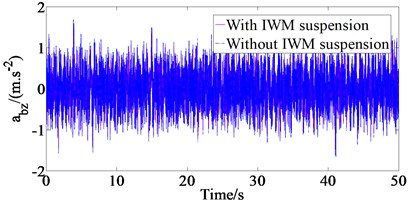a) Case 1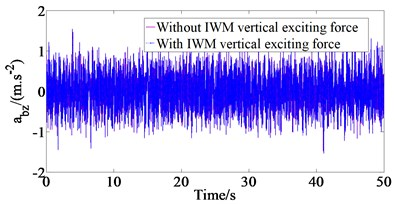b) Case 2

From the results of Fig. 3, the values of ${a}_{wbz}$with IWM suspension system are indicated to reduce by 8.6 %, 8.6 %, 8.5 %, 8.5 % and 8.4 % in comparison to without IWM suspension system when the vehicle moves on changing bad road conditions. This means that the IWM suspension system has significantly improved the EV ride quality.

Comparing with the IWM suspension system and without IWM suspension system when the IWM mass condition changes: the ${a}_{wbz}$ value for five different IWM mass conditions were analyzed when vehicle moves on ISO road surface class B at vehicle speed 50 km/h and IWM vertical exciting force ${F}_{mz0}=1500\mathrm{c}\mathrm{o}\mathrm{s}\left(1000\pi t\right)/N$. The ${a}_{wbz}$ value with the IWM suspension system in comparison to without IWM suspension system for five different IWM mass road surface conditions is shown in Fig. 4. From the results of Fig. 4, the values of ${a}_{wbz}$with IWM suspension system are indicated to reduce by 8.4 %, 8.6 %, 9.7 %, 9.9 % and 9.9 % in comparison to without IWM suspension system when the value of the IWM mass increases. The EV ride quality with the IWM suspension system is significantly improved for the cases of great IWM mass values.

Fig. 3. The ${a}_{wbz}$ value for five different road surface conditions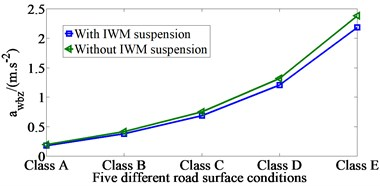Fig. 4. The ${a}_{wbz}$ value for five different IWM mass conditions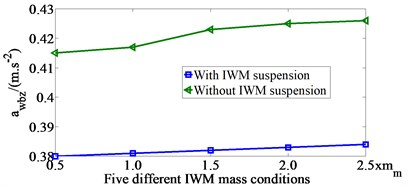#### 4. Conclusions

In this study, a one-quarter EV dynamic model is developed for analyzing the effect of in- IWM suspension system on (EV) ride comfort. The major conclusions drawn from the analysis can be summarized as follows:

1) The value of ${a}_{wbz}$ with IWM suspension system reduces by 8.6 % in comparison to without IWM suspension system which means that the effect of IWM suspension system on the EV ride quality is significant;

2) The values of ${a}_{wbz}$ with IWM suspension system reduce by 8.6 %, 8.6 %, 8.5 %, 8.5 % and 8.4 % in comparison to without IWM suspension system when the vehicle moves on changing bad road conditions;

3) The values of ${a}_{wbz}$ with IWM suspension system reduce by 8.4 %, 8.6 %, 9.7 %, 9.9 % and 9.9 % compared with that without IWM suspension system, when the value of the IWM mass increases.

#### Acknowledgements

The work described in this paper was supported by Thai Nguyen University of Technology for a scientific project (Code: T2018-B26).

1. Qin Y., He C., Shao X., Du H., Xiang C., Dong M. Vibration mitigation for in-wheel switched reluctance motor driven electric vehicle with dynamic vibration absorbing structures. Journal of Sound and Vibration, Vol. 419, 2018, p. 249-267. [Publisher]
2. Abu Bakar S. A., Muhamad Said M. F., Aziz A. A. Ride comfort performance evaluations on electric vehicle conversion via simulations. ARPN Journal of Engineering and Applied Sciences, Vol. 10, Issue 17, 2015, p. 7794-7798. [Search CrossRef]
3. Jin L., Yu Y., Fu Y. Study on the ride comfort of vehicles driven by in-wheel motors. Advances in Mechanical Engineering, Vol. 8, Issue 3, 2016, p. 1-9. [Publisher]
4. Abdussalam Ali A. O., Başar Özkan Analysis of effect of in-wheel electric motors mass on passive and active suspension systems. ARPN Journal of Engineering and Applied Sciences, Vol. 10, Issue 17, 2015, p. 5924-5928. [Search CrossRef]
5. Fangwu Ma, Jiawei Wang, Yang Wang, Longfan Yang Optimization design of semi-active controller for in-wheel motors suspension. Journal of Vibroengineering, Vol. 20, Issue 8, 2018, p. 2908-2924. [Publisher]
6. Liu M., Gu F., Zhang Y. Ride comfort optimization of in-wheel-motor electric vehicles with in-wheel vibration absorbers. Energies, Vol. 10, 2017, p. 1647. [Publisher]
7. Ma Y., Deng Z., Xie D. Control of the active suspension for in-wheel motor. Journal of Advanced Mechanical Design, Systems, and Manufacturing, Vol. 7, Issue 4, 2013, p. 535-543. [Publisher]
8. Tan Di, Lu Chao, Zhang Xueyi Dual-loop PID control with PSO algorithm for the active suspension of the electric vehicle driven by in-wheel motor. Journal of Vibroengineering, Vol. 18, Issue 6, 2016, p. 3915-3929. [Publisher]
9. Yu Yuewei, Zhao Leilei, Zhou Changcheng Influence of rotor-bearing coupling vibration on dynamic behavior of electric vehicle driven by in-wheel motor. IEEE Access, Vol. 7, 2019, p. 63540-63549. [Publisher]
10. Tan D., Wang Q., Wu Y. Modal analysis of in-wheel motor-driven electric vehicle based on bond graph theory. Shock and Vibration, Vol. 2017, 2017, p. 6459154. [Publisher]
11. ISO 2631-1. Mechanical Vibration and Shock-Evaluation of Human Exposure to Whole-Body Vibration, Part I: General Requirements. The International Organization for Standardization, 1997. [Search CrossRef]
12. Long L. X., Quynh L. V., Cuong B. V. Study on the influence of bus suspension parameters on ride comfort. Vibroengineering Procedia, Vol. 21, 2018, p. 77-82. [Publisher]
13. Le V. Q., Zhang J., Liu X., Wang Y. Nonlinear dynamic analysis of interaction between vehicle and road surfaces for 5-axle heavy truck. Journal of Southeast University, Vol. 27, Issue 4, 2011, p. 405-409. [Search CrossRef]
14. Dodds C. J., Robson J. D. The description of road surface roughness. Journal of Sound and Vibration, Vol. 31, Issue 2, 1973, p. 175-183. [Publisher]
15. Pham Thi Thanh Dung Study on the Effects of Design Parameters of the Vehicle Suspension System on Vehicle Ride Comfort. Master of Science Thesis, Thai Nguyen University of Technology, Thai Nguyen, Vietnam, 2019. [Search CrossRef]
16. ISO 8068. Mechanical Vibration-Road Surface Profiles-Reporting of Measured Data. International Organization for Standardization, 1995. [Search CrossRef]

#### Cited By

 Lecture Notes in Networks and Systems Le Van Quynh, Bui Van Cuong, Hoang Anh Tan, Canh Chi Huan 2023 Processes Dongfang Song, Hong Ji, Kang Li 2022 SAE Technical Paper Series Mostafa I. Yacoub, Mostafa Sh. Asfoor 2021 Journal of Mechanical Engineering, Automation and Control Systems Shuting Huang, Vanliem Nguyen 2021 Vibroengineering PROCEDIA Peiling Wang 2020